Constructing a right triangle when hypotenuse and side is given (RHS)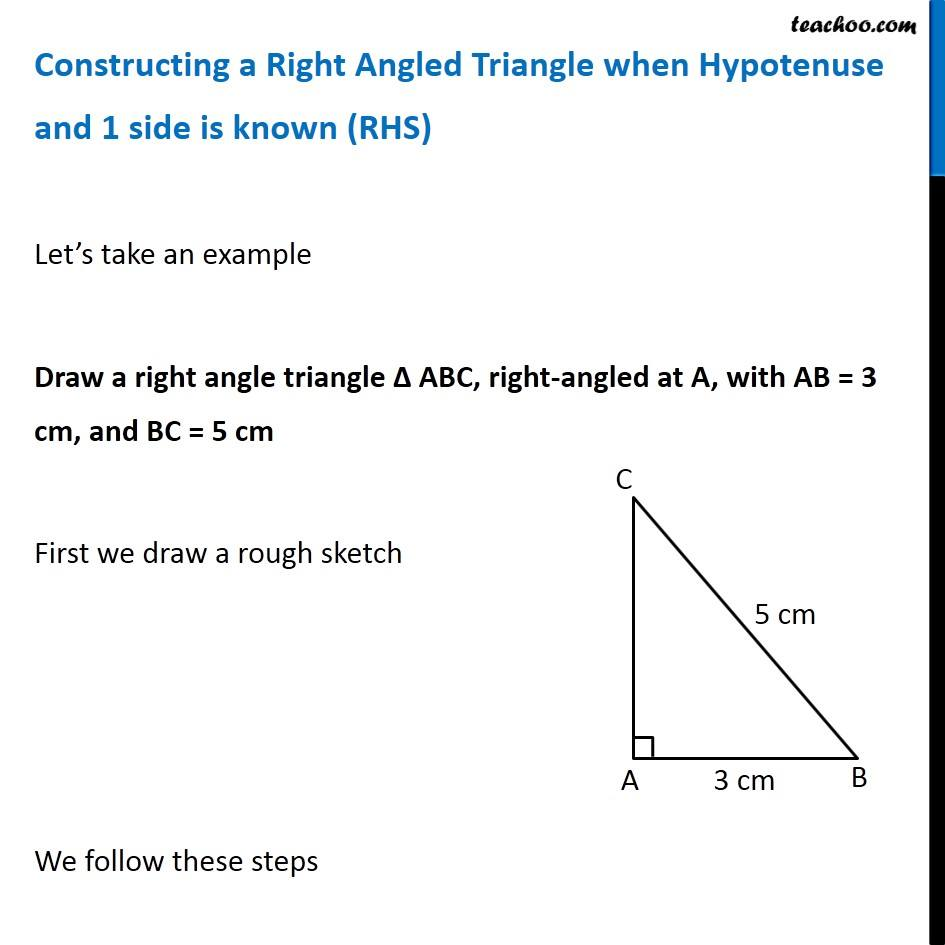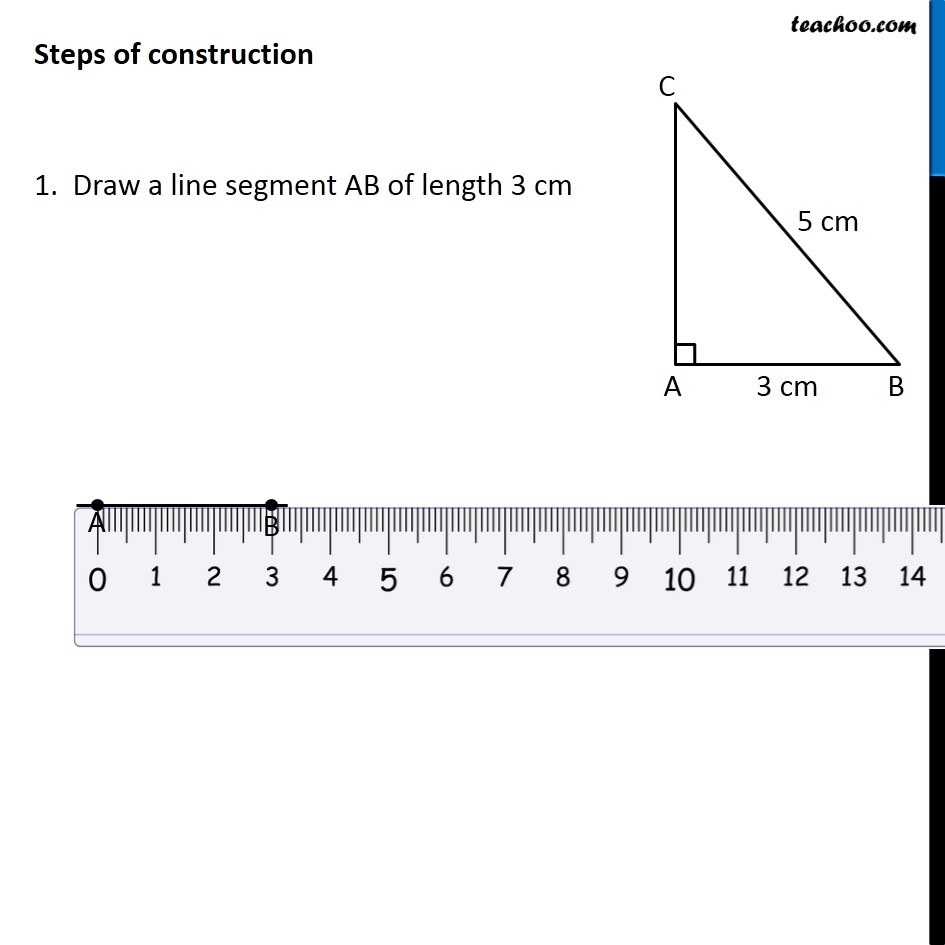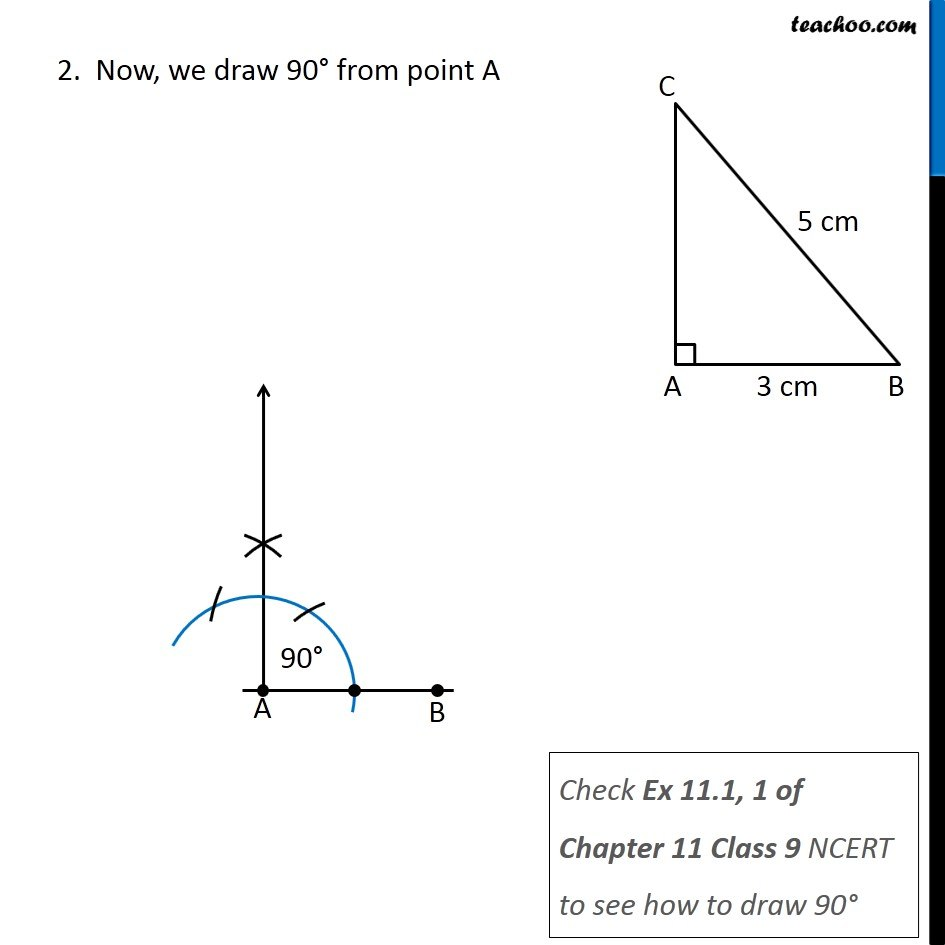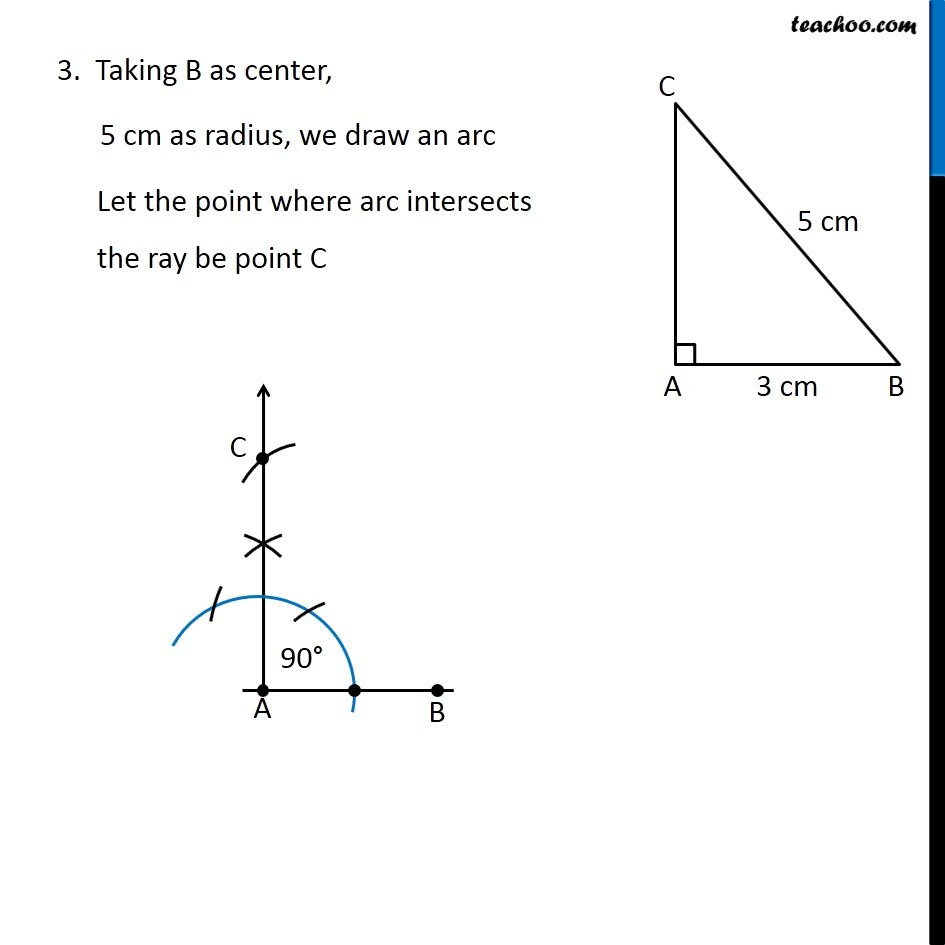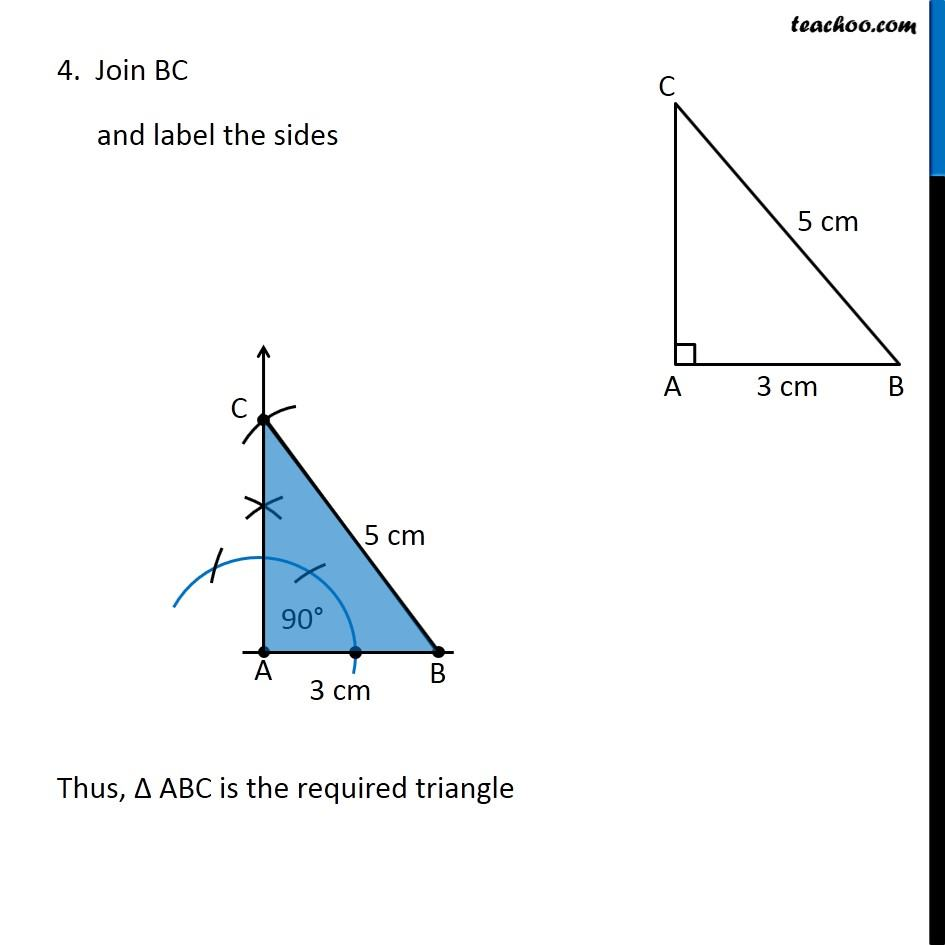Learn in your speed, with individual attention - Teachoo Maths 1-on-1 Class

### Transcript

Constructing a Right Angled Triangle when Hypotenuse and 1 side is known (RHS) Let’s take an example Draw a right angle triangle Δ ABC, right-angled at A, with AB = 3 cm, and BC = 5 cm First we draw a rough sketch We follow these steps Steps of construction 1. Draw a line segment AB of length 3 cm 2. Now, we draw 90° from point A Check Ex 11.1, 1 of Chapter 11 Class 9 NCERT to see how to draw 90° 3. Taking B as center, 5 cm as radius, we draw an arc Let the point where arc intersects the ray be point C 4. Join BC and label the sides Thus, Δ ABC is the required triangle